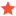• 0 Vote(s) - 0 Average
• 1
• 2
• 3
• 4
• 5
 Kuka Axis-Angle to PosedsitnikJunior MemberPosts: 3 Threads: 3 Joined: Aug 2019 Reputation: 0 12-11-2019, 09:47 AM Hi, I would like to use online programming for moving a robot from one point to another. Let's say I have N points in the array where every point is coded as a 6-axis-angle (\$AXIS_ACT Kuka system variable). Is there any way to directly convert this angles to the robot pose matrix (as robot.Pose())? Thank you in advance! Find ReplyAlbertModeratorPosts: 922 Threads: 1 Joined: Apr 2018 Reputation: 45 12-11-2019, 07:25 PM Yes, this is possible. You can use SolveFK to calculate the forward kinematics (robot pose given robot joints) and SolveIK to calculate inverse kinematics (robot joints given the robot pose. Example: Code:```robot_joints = [...] # robot axis such as AXIS_ACT # Calculate the robot flange with respect to the robot base flange_pose = robot.SolveFK(robot_joints) # Calculate the pose of the active tool with respect to the active reference frame target_pose = robot.SolveFK(robot_joints, robot.PoseTool(), robot.PoseFrame()) # Convert the pose to KUKA XYZABC format (6D array/list): kuka_xyzabc = Pose_2_KUKA(target_pose)``` Find Reply

 Users browsing this thread: 1 Guest(s)